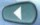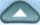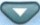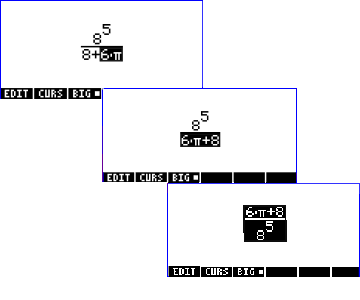HP49 Equation Writer
HP49 Equation Writer

HP49 Equation WriterHP49 Equation Writer is one of the most powerfull resourses of HP49. It permits write complexes equations as you write them in the paper. You can write symbolic and numerical expressions. HP49 also offers option to expand binomial expressions and see previos expressions you put in the stack.
 Symbolic expression can easily be written in HP49 equation writer. The softkey BIG, actived by F3, permits to see the equation in two sizes, BIG or short.How can I work with HP49 Equation Writer?
 The Equation Writer can be accessed by the keyand can be easily operated.You just need to type the expression and use the arrow keys.For example, in the picture at left, when the expression 6.p is shaded, it means you are able to work with it and can sum, divide, multiply it or to do other operations. To do it just press some of the operators (+, -, / , x, log, y^x). HP49 inserts parentesis "( )", automatically, when needed.

In the HP49 Equation Writer, sometimes, a part of the equation shaded (painted of black). It means that it is the part of equation you can work on it. Press arrow keys to mark the equation and
the mathematical operator to continue.

 This resourse of HP49 Equation Writer permits you know what is the part of the equation that you are working on it. Use arrow keys, go back or forward, up or down to correct the equation or also insert new elements. The command EDIT permits you to correct the part of the equation shaded (painted of black).

 Cursor
 The softkey CURS puts the cursor in the part of the equation you wish.   It can be used to easily go to a part of the equation that needs to be edited.   Press [F2], to access it and [EXE] to exit.